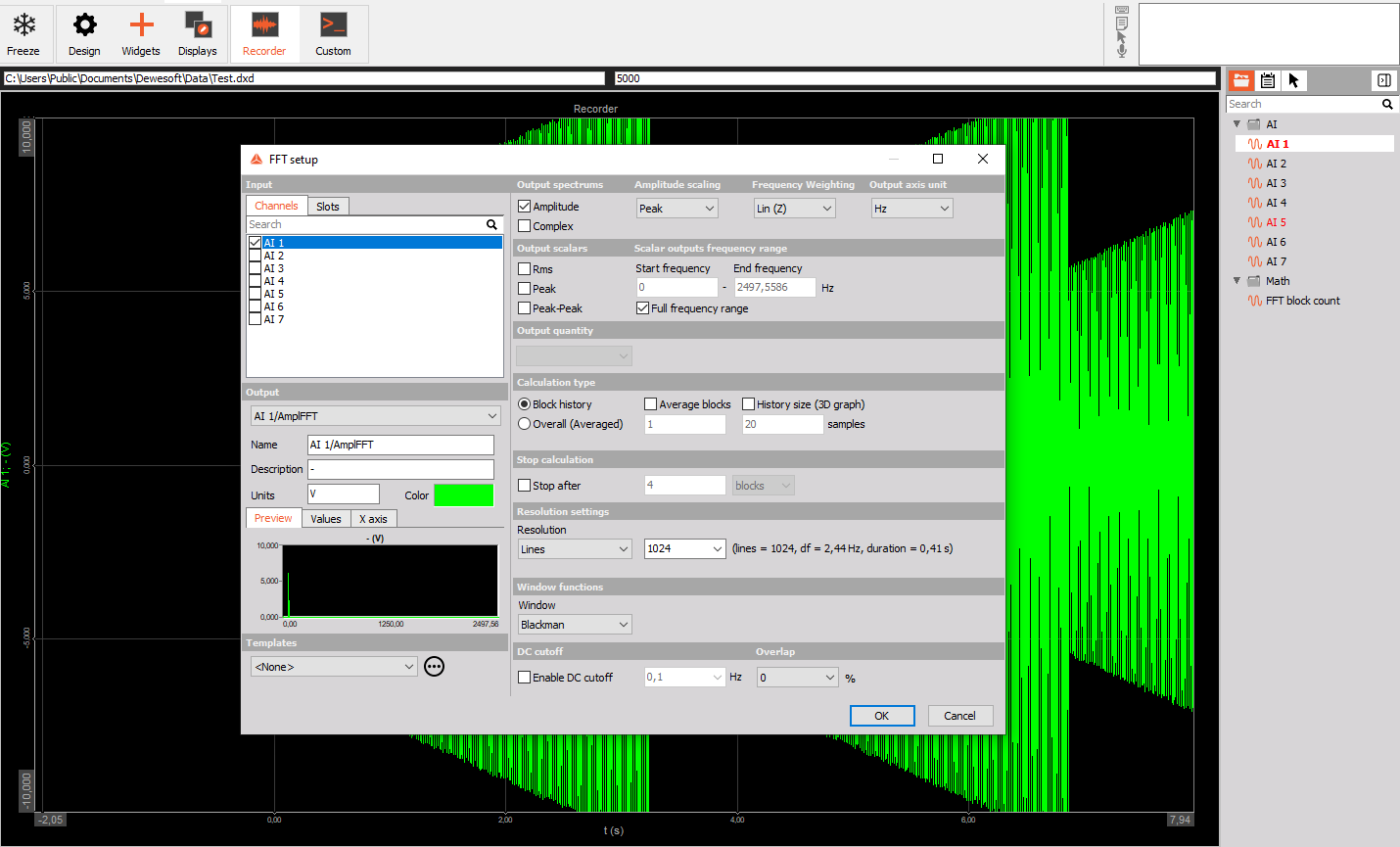# Math

Dewesoft has many different data acquisition sources. Taking raw data measurement is often not enough to come to the wanted result. Data processing is one of the most important features to be able to recalculate (Formula editor) signals, filtering them or make other signal processing. The following section gives an overview of basic mathematical modules.

## Enabling Mathematics and Filter

First you have to make sure that the mathematics module is enabled. On the Dewesoft Setup screen next to the Analog and the other available tabs, a Math tab has to be displayed. Otherwise please refer to Add module to enable the mathematics functions.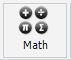## Math setup screen

When we press the math tab a setup screen is opened. Here different modules can be added.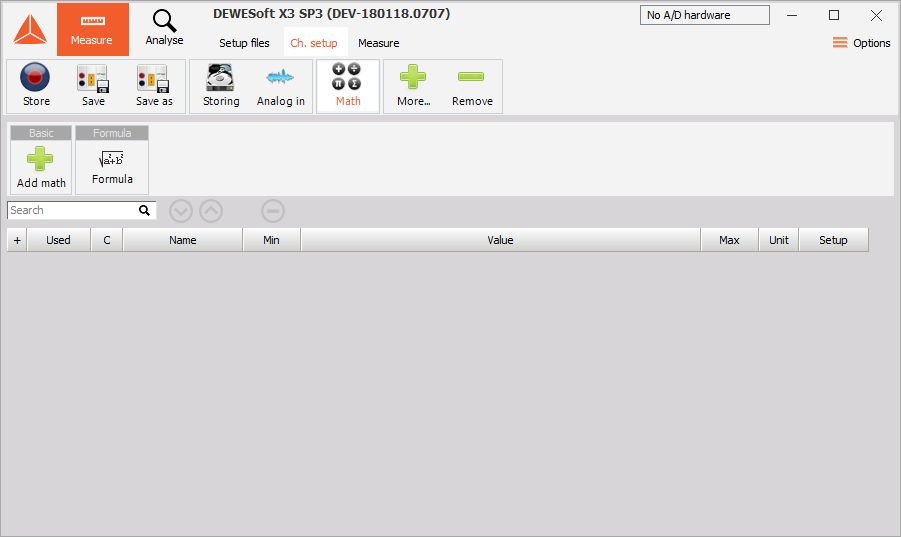There are also three function keys:

• Remove selected mathematical function or filter. If you want to delete the channel, simply select desired filter or function with a mouse click and then press this minus button above the channels.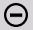• Move UP selected function within the list. If you want to move the channel upwards, simply select the desired filter or function with a mouse click and then press this button above the channels. For the first channel in the list this button is not available.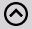• Move DOWN selected function within the list. If you want to move the channel downwards, simply select the desired filter or function with a mouse click and then press this button above the channels. For the last channel in the list this button is not available.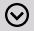Moving the modules up and down doesn’t change the calculation order. Dewesoft automatically detects the references and orders the mathematical channels. So, in general, we can first select a filter on formula channel and after that define this formula channel. Dewesoft will then first calculate the formula and after that calculate the filter.

For extensive calculation, Dewesoft offers the possibility to calculate formulas and filters in separate CPU’s. This is available only on multi-core processors. It will work best if the math modules are not referencing each other, for example that we calculate the IIR filter of several input channels. Then the calculation can be nicely split into several cores. If the channels are referencing each other, this is not possible since the order of calculation is strict and well known.

## Adding Math to setup screen

On the Dewesoft Math setup screen, you can add new mathematics by left clicking on Add math module.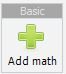A list of all functions appears: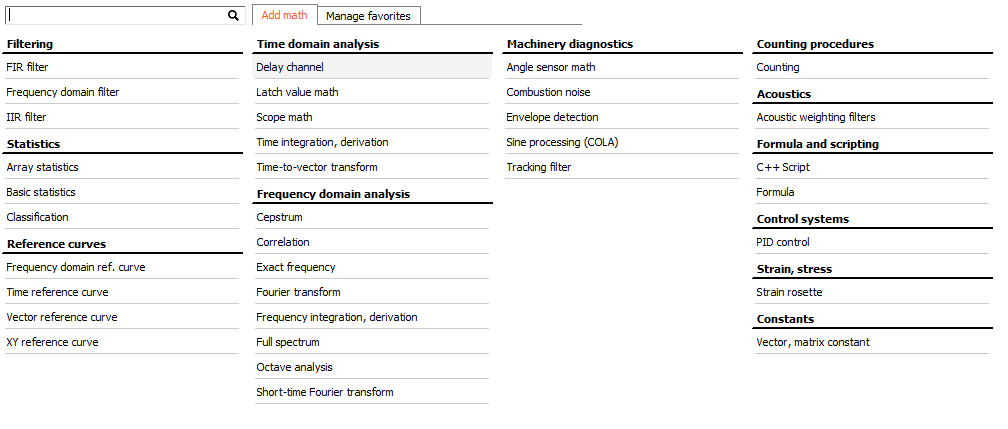To add a function to the Dewesoft Math setup screen just click on desired function from the list or search for it in the search window: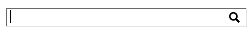## Add multiple math modules at once

Right click on the selected math module and select Add multiple option.

Mutliple maths can be added in all the all maths view or in the favourites view.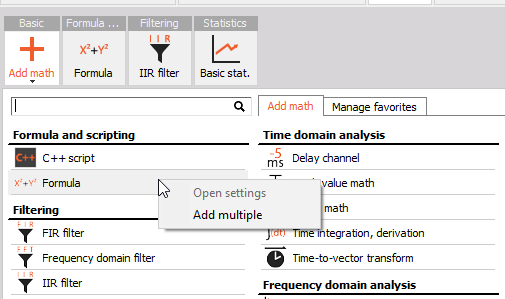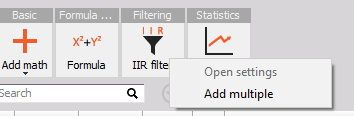When the option is selected, a menu appears where you define the wanted number of math modules.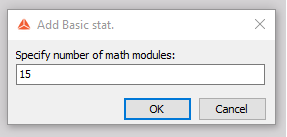## Math in measure

Mathematical function can be applied to channels during the measurement mode.

Right click on the channel on which you want to perform mathematical operation and select it from the menu.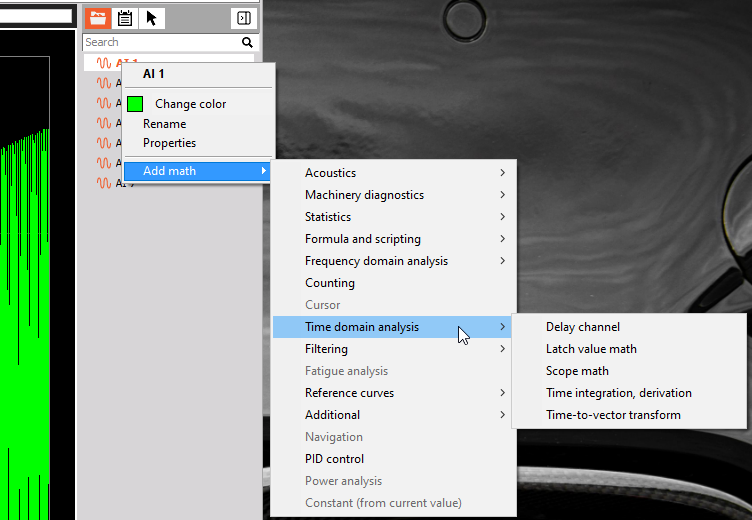For example, if we want to add an FFT, we click on the channel with the right mouse button, select Add math -> Frequency domain analysis -> FFT.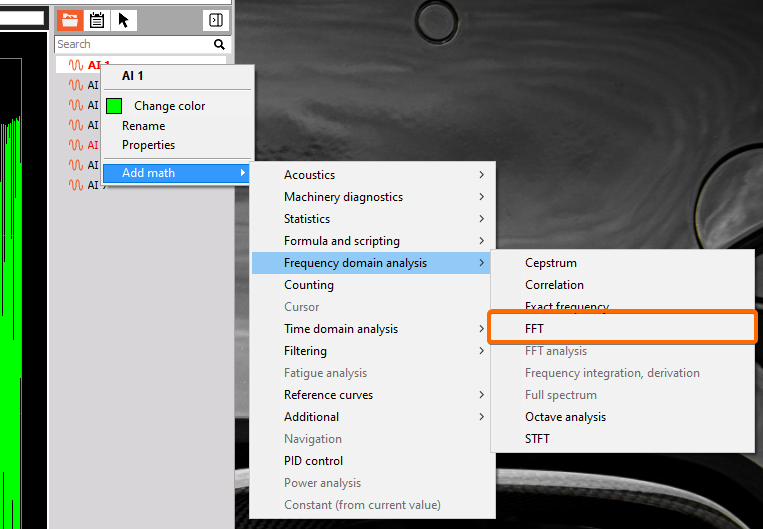When the FFT is added, a setup for FFT appears.